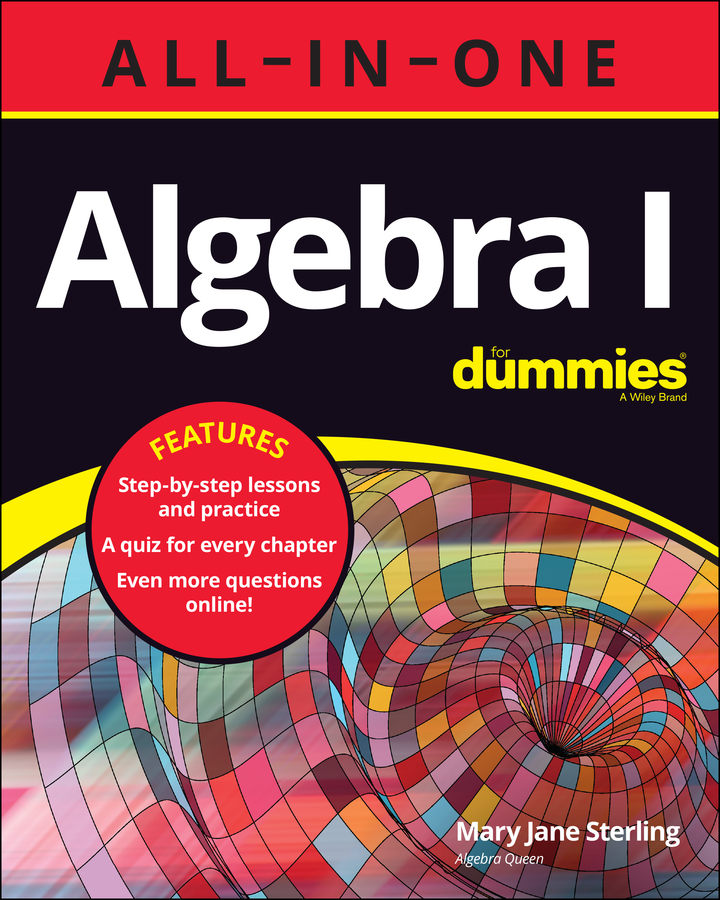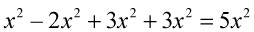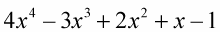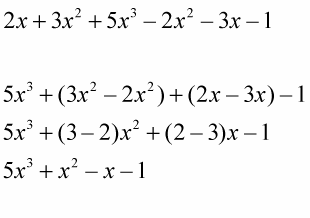##### Algebra I All-in-One For DummiesTo add or subtract with powers, both the variables and the exponents of the variables must be the same. You perform the required operations on the coefficients, leaving the variable and exponent as they are.

When adding or subtracting with powers, the terms that combine always have exactly the same variables with exactly the same powers. These rules are true for multiplying and dividing exponents as well.

Example 1:

x + x + x = 3x

Because the variables are the same (x) and the powers are the same (there are no exponents, so the exponents must be 1), you can add the variables.

Example 2:Because the variables are the same (x) and the powers are the same (2), you can perform the required operations on the variables.

Example 3:Although the variables are the same (x), the powers are not the same (1, 2, 3, and 4). You can't simplify these terms because only the variables are the same, and both the variables and the powers need to be the same.

Example 4:Sometimes not all of the variables and powers will be the same — you may encounter a problem that has several groups of variables and powers that are not the same. In this case, you only add or subtract terms whose variables and powers are the same. (Notice that the exponents are listed in order from highest to lowest. This is a common practice to make answers easy to compare.)